# initial

System response to initial states of state-space model

## Syntax

``[y,tOut] = initial(sys,xinit)``
``[y,tOut] = initial(sys,xinit,tFinal)``
``[y,tOut] = initial(sys,xinit,[t0,tFinal])``
``[y,tOut] = initial(sys,xinit,t)``
``[y,tOut] = initial(sys,xinit,t,p)``
``[y,tOut] = initial(sys,{xinit,pinit},t,p)``
``[y,tOut,x] = initial(sys,xinit)``
``[y,tOut,x,pOut] = initial(sys,xinit,t,p)``
``initial(sys,xinit,___)``

## Description

For state-space and sparse state-space models, `initial` computes the unforced system response y to initial states xinit.

• Continuous time:

`$\begin{array}{cc}\stackrel{˙}{x}=Ax,& x\left({t}_{0}\right)={x}_{init}\\ y=Cx& \end{array}$`
• Discrete time:

`$\begin{array}{cc}x\left[k+1\right]=Ax\left[k\right]& x\left[{k}_{0}\right]={x}_{init}\\ y=Cx\left[k\right]& \end{array}$`

For linear time-varying or linear parameter-varying state-space models, `initial` computes the response with initial state xinit, initial parameter pinit (LPV models), and input held to the offset value (u(t) = u0(t) or u(t) = u0(t,p). This corresponds to the initial condition response of the local linear dynamics.

````[y,tOut] = initial(sys,xinit)` computes the unforced initial response `y` from the initial state `xinit`. The time vector `tOut` is in the time units of `sys`. `initial` automatically determines the time steps and duration of the simulation based on the system dynamics.```
````[y,tOut] = initial(sys,xinit,tFinal)` simulated response from `t = 0` to the final time ```t = tFinal```. The function uses system dynamics to determine the intervening time steps.```
````[y,tOut] = initial(sys,xinit,[t0,tFinal])` simulates the response from `t0` to `tFinal`. (since R2023b)```
````[y,tOut] = initial(sys,xinit,t)` returns the initial response of `sys` at the times specified in the vector `t`.```
````[y,tOut] = initial(sys,xinit,t,p)` also specifies the parameter trajectory `p` for LPV models. (since R2023a)```

example

````[y,tOut] = initial(sys,{xinit,pinit},t,p)` also specifies the initial parameter value and parameter trajectory `p` for LPV models. `pinit` is required for implicit parameter trajectories. (since R2023a)```

example

````[y,tOut,x] = initial(sys,xinit)` returns the output response `y`, the time vector `tOut` and the state trajectories `x`. The array `y` has as many rows as time samples (length of `tOut`) and as many columns as outputs. Similarly, `x` has `length(tOut)` rows and as many columns as states.```
````[y,tOut,x,pOut] = initial(sys,xinit,t,p)` also returns parameter trajectories `pOut`, when `sys` is an `lpvss` model. (since R2023a)```
````initial(sys,xinit,___)` plots the initial response of `sys`. This syntax is equivalent to `initialplot(sys,__)`. When you need additional plot customization options, use `initialplot` instead.```

## Examples

collapse all

For this example, generate a random state-space model with 5 states and create the plot for the system response to the initial states.

```rng("default") sys = rss(5); x0 = [1,2,3,4,5]; initial(sys,x0)```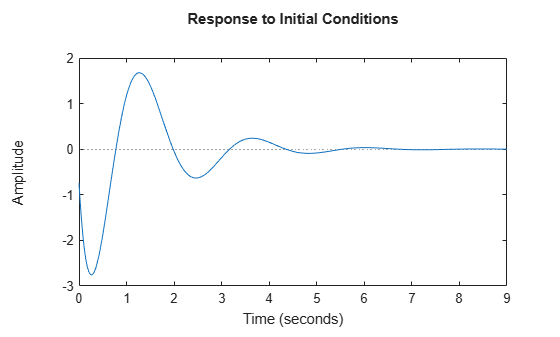Plot the response of the following state-space model:

`$\begin{array}{rcl}\left[\begin{array}{l}{\underset{}{\overset{˙}{x}}}_{1}\\ {\underset{}{\overset{˙}{x}}}_{2}\end{array}\right]& =& \left[\begin{array}{cc}-0.5572& -0.7814\\ 0.7814& 0\end{array}\right]\left[\begin{array}{l}{x}_{1}\\ {x}_{2}\end{array}\right]\\ y& =& \left[\begin{array}{cc}1.9691& 6.4493\end{array}\right]\left[\begin{array}{l}{x}_{1}\\ {x}_{2}\end{array}\right].\end{array}$`

Take the following initial condition:

`$x\left(0\right)=\left[\begin{array}{l}1\\ 0\end{array}\right].$`

```a = [-0.5572, -0.7814; 0.7814, 0]; c = [1.9691 6.4493]; x0 = [1 ; 0]; sys = ss(a,[],c,[]); initial(sys,x0)```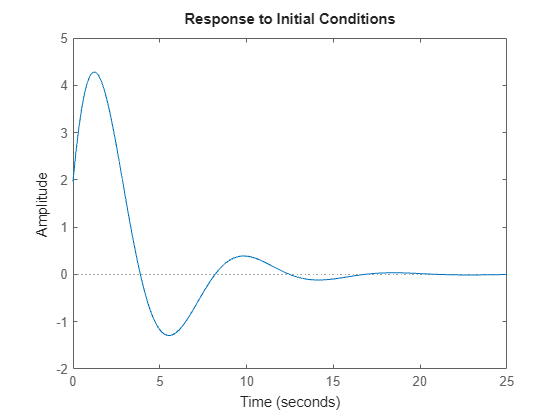Consider the following two-input, two-output dynamic system.

`$sys\left(s\right)=\left[\begin{array}{cc}0& \frac{3s}{{s}^{2}+s+10}\\ \frac{s+1}{s+5}& \frac{2}{s+6}\end{array}\right].$`

Convert the `sys` to state-space form since initial condition response plots are supported only for state-space models.

```sys = ss([0, tf([3 0],[1 1 10]) ; tf([1 1],[1 5]), tf(2,[1 6])]); size(sys)```
```State-space model with 2 outputs, 2 inputs, and 4 states. ```

The resultant state-space model has four states. Hence, provide an initial condition vector with four elements.

`x0 = [0.3,0.25,1,4];`

Create the initial condition response plot.

`initial(sys,x0);`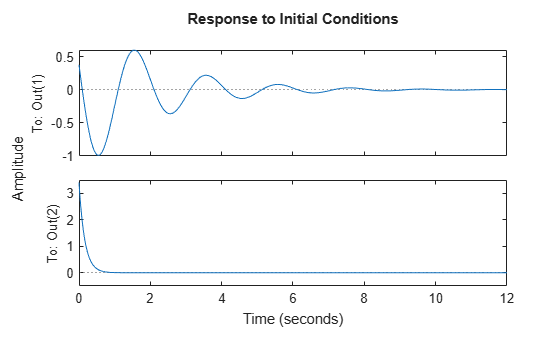The resultant plot contains two subplots - one for each output in `sys`.

For this example, examine the initial condition response of the following zero-pole-gain model and limit the plot to `tFinal` = 15 s.

First, convert the `zpk` model to an `ss` model since `initial` only supports state-space models.

```sys = ss(zpk(-1,[-0.2+3j,-0.2-3j],1)*tf([1 1],[1 0.05])); tFinal = 15; x0 = [4,2,3];```

Now, create the initial conditions response plot.

`initial(sys,x0,tFinal);`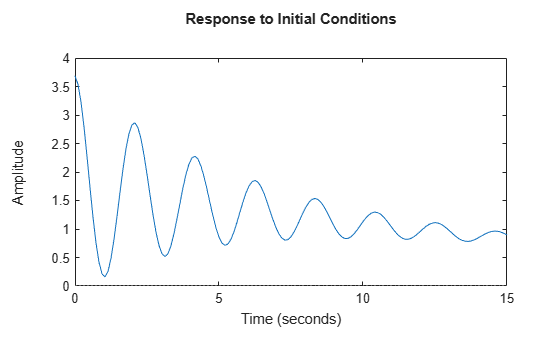For this example, plot the initial condition responses of three dynamic systems.

First, create the three models and provide the initial conditions. All the models should have the same number of states.

```rng('default'); sys1 = rss(4); sys2 = rss(4); sys3 = rss(4); x0 = [1,1,1,1];```

Plot the initial condition responses of the three models using time vector `t` that spans 5 seconds.

```t = 0:0.1:5; initial(sys1,'r--',sys2,'b',sys3,'g-.',x0,t)```Extract the initial condition response data of the following state-space model with two states:

`$\begin{array}{rcl}\left[\begin{array}{l}{\underset{}{\overset{˙}{x}}}_{1}\\ {\underset{}{\overset{˙}{x}}}_{2}\end{array}\right]& =& \left[\begin{array}{cc}-0.5572& -0.7814\\ 0.7814& 0\end{array}\right]\left[\begin{array}{l}{x}_{1}\\ {x}_{2}\end{array}\right]\\ y& =& \left[\begin{array}{cc}1.9691& 6.4493\end{array}\right]\left[\begin{array}{l}{x}_{1}\\ {x}_{2}\end{array}\right].\end{array}$`

Use the following initial conditions:

`$x\left(0\right)=\left[\begin{array}{l}1\\ 0\end{array}\right].$`

```a = [-0.5572, -0.7814; 0.7814, 0]; c = [1.9691 6.4493]; x0 = [1 ; 0]; sys = ss(a,[],c,[]); [y,tOut,x] = initial(sys,x0);```

The array `y` has as many rows as time samples (length of `tOut`) and as many columns as outputs. Similarly, `x` has rows equal to the number of time samples (length of `tOut`) and as many columns as states.

For this example, extract the initial condition response data of a state-space model with 6 states, 3 outputs and 2 inputs.

First, create the model and provide the initial conditions.

```rng('default'); sys = rss(6,3,2); x0 = [0.1,0.3,0.05,0.4,0.75,1];```

Extract the initial condition responses of the model using time vector `t` that spans 15 seconds.

```t = 0:0.1:15; [y,tOut,x] = initial(sys,x0,t);```

The array `y` has as many rows as time samples (length of `tOut`) and as many columns as outputs. Similarly, `x` has rows equal to the number of time samples (length of `tOut`) and as many columns as states.

For this example, `throttleLPV.m` that defines the dynamics of a nonlinear engine throttle which behaves linearly in the 15 degrees to 90 degrees opening range.

Use `lpvss` to create the model. This model is parameterized by the throttle angle, which is the first state of the model.

```c0 = 50; k0 = 120; K0 = 1e4; b0 = 4e4; yf = 15*K0/(k0+K0); Ts = 0; sys = lpvss("x1",@(t,p) throttleLPV(p,c0,k0,b0,K0),Ts,0,15);```

You can compute the initial response for this model along a trajectory $\mathit{p}\left(\mathit{t}\right)$.

Compute the response when you start at the lower end of linear range with a small angular velocity.

```pFcn = @(t,x,u)x(1); x0 = [15;10]; p0 = x0(1); t = linspace(0,0.6,500); initial(sys,{x0,p0},t,pFcn)```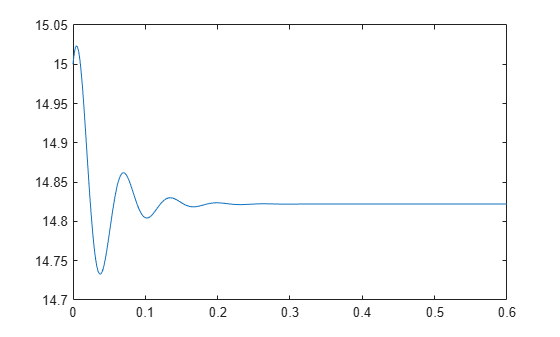Compute the response when you start at the lower end of linear range with enough angular velocity to hit the upper end of this range.

```x0 = [15;5e3]; p0 = x0(1); t = linspace(0,1,1000); initial(sys,{x0,p0},t,pFcn)```View the data function.

`type throttleLPV.m`
```function [A,B,C,D,E,dx0,x0,u0,y0,Delays] = throttleLPV(x1,c,k,b,K) % LPV representation of engine throttle dynamics. % Ref: https://www.mathworks.com/help/sldo/ug/estimate-model-parameter-values-gui.html % x1: scheduling parameter (throttle angle; first state of the model) % c,k,b,K: physical parameters A = [0 1; -k -c]; B = [0; b]; C = [1 0]; D = 0; E = []; Delays = []; x0 = []; u0 = []; y0 = []; % Nonlinear displacement value NLx = max(90,x1(1))-90+min(x1(1),15)-15; % Capture the nonlinear contribution as a state-derivative offset dx0 = [0;-K*NLx]; ```

## Input Arguments

collapse all

Dynamic system, specified as a SISO or MIMO dynamic system model or array of dynamic system models. You can only use state-space models of the following types:

• Continuous-time or discrete-time numeric `ss` models.

• Generalized or uncertain LTI models such as `genss` or `uss` models. (Using uncertain models requires Robust Control Toolbox™ software.)

• For tunable control design blocks, the function evaluates the model at its current value for both plotting and returning response data.

• For uncertain control design blocks, the function plots the nominal value and random samples of the model. When you use output arguments, the function returns response data for the nominal model only.

• Sparse state-space models such as `sparss` and `mechss` models. You must specify final time `tFinal` for sparse state-space models.

• Linear time-varying (`ltvss`) and linear parameter-varying (`lpvss`) models.

If `sys` is an array of models, the function plots the responses of all models in the array on the same axes.

End time to compute the response, specified as a positive scalar value. `initial` simulates the response from `t = 0` to `t = ``tFinal`.

• For continuous-time systems, the function determines the step size and number of points automatically from system dynamics. Express `tFinal` in the system time units, specified in the `TimeUnit` property of `sys`.

• For discrete-time systems, the function uses the sample time of `sys` as the step size. Express `tFinal` in the system time units, specified in the `TimeUnit` property of `sys`.

• For discrete-time systems with unspecified sample time (```Ts = -1```), `initial` interprets `tFinal` as the number of sampling periods to simulate.

Since R2023b

Time range, specified as a two-element vector of positive scalar values. `initial` simulates the response from `t = t0` to `t = tFinal`.

Time vector at which to compute the response, specified as a vector of positive scalar values. Express `t` in the system time units, specified in the `TimeUnit` property of `sys`.

• For continuous-time models, specify `t` in the form `Ti:dt:Tf`. To obtain the response at each time step, the function uses `dt` as the sample time of a discrete approximation to the continuous system.

• For discrete-time models, specify `t` in the form `Ti:Ts:Tf`, where `Ts` is the sample time of `sys`.

Initial condition of the states, specified as a vector. `xinit` must have the same length as the number of states in `sys`.

Since R2023b

Parameter trajectory of the LPV model, specified as a matrix or a function handle.

• For exogenous or explicit trajectories, specify `p` as a matrix with dimensions N-by-Np, where N is the number of time samples and Np is the number of parameters.

Thus, the row vector `p(i,:)` contains the parameter values at the ith time step.

• For endogenous or implicit trajectories, specify `p` as a function handle of the form p = F(t,x,u) in continuous time and p = F(k,x,u) in discrete time that gives parameters as a function of time t or time sample k, state x, and input u. The initial parameter value `pinit` is required for this input method.

Since R2023b

Initial value of the parameters of LPV model, specified as a vector. `pinit` must have the same length as the number of parameters in `sys`.

## Output Arguments

collapse all

Response data, returned as an array.

• For SISO systems, `y` is a column vector of the same length as `t` (if provided) or `tOut` (if you do not provide `t`).

• For single-input, multi-output systems, `y` is a matrix with as many rows as there are time samples and as many columns as there are outputs. Thus, the jth column of `y`, or `y(:,j)`, contains the response of from the input to the jth output.

• For MIMO systems, the dimensions of `y` are then N-by-Ny, where:

• N is the number of time samples.

• Ny is the number of system outputs.

Times at which response is computed, returned as a vector. When you do not provide a specific vector `t` of times, `initial` chooses this time vector based on the system dynamics. The times are expressed in the time units of `sys`.

State trajectories, returned as an array. `x` contains the evolution of the states of `sys` at each time in `t` or `tOut`. The dimensions of `x` are N-by-Nx, where:

• N is the number of time samples.

• Nx is the number of states.

Parameter trajectories, returned as an array. When `sys` is a linear-parameter varying (`lpvss`) model, `pOut` contains the evolution of the parameters of `sys`. The dimensions of `pOut` are N-by-Np, where:

• N is the number of time samples.

• Np is the number of parameters.

## Version History

Introduced before R2006a

expand all